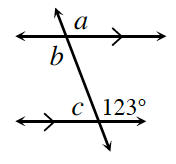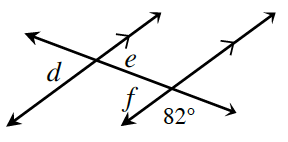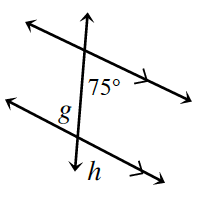### Home > CCG > Chapter 6 > Lesson 6.1.4 > Problem6-34

6-34.

Use your theorems about parallel lines and the angles formed with a third line to find the measures of the labeled angles below. Show each step you use and be sure to justify each one with an angle theorem from your Angle Relationships Toolkit. Be sure to write down your reasoning in the order that you find the angles.

1.There are multiple correct ways to find the angle measures.

If parallel lines are cut by a transversal, then
corresponding angles have equal measures.

Vertical angles must also have equal measures.

The $123º$ angle corresponds to which angle?

$m∠a=123º$ because corresponding angles on parallel lines have equal measures.

$m∠a=m∠b$ because vertical angles have equal measures.

$m∠c+123º=180º$ because they are supplementary angles.

So what is $m∠c$?

$m∠a=123º$, $m∠b=123º$, $m∠c=57º$

1.$m∠d=98º$, $m∠e=98º$, $m∠f=98º$

Make sure you write down your reasoning.

1.